Related Articles

# Class 9 RD Sharma Solutions – Chapter 16 Circles- Exercise 16.2

• Last Updated : 12 May, 2021

### Question 1. The radius of a circle is 8 cm and the length of one of its chords is 12 cm. Find the distance of the chord from the centre.

Solution: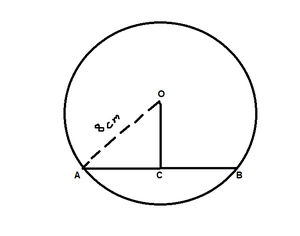According to the question, given that

Chord (AB) = 12cm

Find: the distance of the chord from the centre, i.e., OC

So, draw a perpendicular OC on AB.

As we know that, perpendicular from centre to chord bisects the chord

So, AC = BC = 12/2 = 6 cm

Now in ΔOCA,

By using Pythagoras theorem,

OA2 = AC2 + OC2

64 = 36 + OC2

OC2 = 64 – 36 = 28

OC = √28

OC = 5.291 (approx.)

Hence, the distance of the chord from the centre is 5.291 cm.

### Question 2. Find the length of a chord which is at a distance of 5 cm from the centre of a circle of radius 10 cm.

Solution: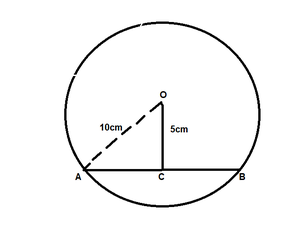According to the question, given that

Distance of the chord from the centre(OC) = 5 cm

Find: the length of a chord AB

So, In ΔOCA,

By using Pythagoras theorem,

OA2 = AC2 + OC2

100 = AC2 + 25

AC2 = 100 – 25 = 75

AC = √75 = 8.66

As we know that, perpendicular from centre to chord bisects the chord

So, AC = BC = 8.66 cm

=> AB = AC + BC = 8.66 + 8.66 = 17.32

Hence, the length of chord AB is 17.32 cm

### Question 3. Find the length of a chord which is at a distance of 4 cm from the centre of a circle of radius 6 cm.

Solution: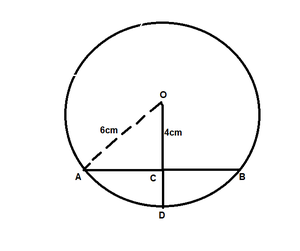According to the question, given that

Distance of the chord from the centre(OC) = 4 cm

Find: the length of a chord, i.e., AB

So, in ΔOCA,

By using Pythagoras theorem,

OA2 = AC2 + OC2

36 = AC2 + 16

AC2 = 36 – 16 = 20

AC = √20 = 4.47

AC = 4.47cm

As we know that, perpendicular from centre to chord bisects the chord

So, AC = BC = 4.47 cm

=> AB = AC + BC = 4.47 + 4.47 = 8.94

Hence, the length of chord AB is 8.94 cm

### Question 4. Two chords AB, CD of lengths 5 cm, 11 cm respectively of a circle are parallel. If the distance between AB and CD is 3 cm, find the radius of the circle.

Solution: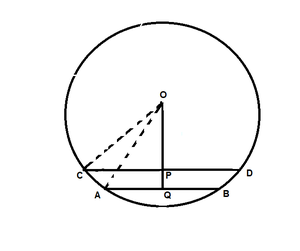According to the question, given that

The length of chord AB = 5 cm

The length of chord CD = 11 cm

PQ = 3 cm

Find: the radius of the circle, i.e., r

So, draw perpendiculars OP on CD and OQ on AB

Let us assume OP = x cm and OC = OA = r cm

As we know that, perpendicular from centre to chord bisects the chord

OP⊥CD,

So, CP = PD = 11/2 cm

And OQ⊥AB

So, AQ = BQ = 5/2 cm

Now, in ΔOCP,

By using Pythagoras theorem,

OC2 = OP2 + CP2

r2 = x2 + (11/2) 2 …..(1)

In ΔOQA,

By using Pythagoras theorem,

OA2 = OQ2 + AQ2

r2 = (x + 3)2 + (5/2)2 …..(2)

From equations (1) and (2), we get

(x + 3)2 + (5/2)2 = x2 + (11/2)2

x2 + 6x + 9 + 25/4 = x2 + 121/4

By using identity, (a + b)2 = a2 + b2 + 2ab

6x = 121/4 – 25/4 − 9

6x = 15

or x = 15/6 = 5/2

Now, substitute the value of x in equation (1), we get

r2 = (5/2)2 + (11/2) 2

r2 = 25/4 + 121/4

r2 = 146/4

r = √146/4 cm

Hence, the radius of the circle is √146/4 cm

### Question 5. Give a method to find the centre of a given circle.

Solution: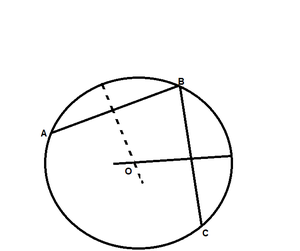Steps of Construction:

Step 1: Let us assume three points, i.e., A, B and C on a circle.

Step 2: Join AB and BC.

Step 3: Now draw perpendicular bisectors of chord AB and BC that intersect each other at a point O.

Step 4: As we know that the perpendicular bisectors of chord always pass through the centre, so the centre of the circle is point O.

### Question 6. Prove that the line joining the mid-point of a chord to the centre of the circle passes through the mid-point of the corresponding minor arc.

Solution: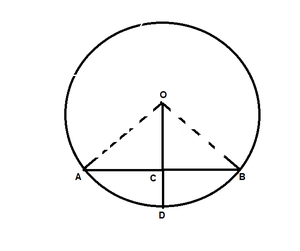According to the question,

Prove: D is the mid-point of arc AB.

Proof:

From the given figure,

Let us assume C is the mid-point of chord AB.

Now, In ΔOAC and ΔOBC

OA = OB [Radius of circle]

OC = OC [Common]

AC = BC [C is the mid-point of chord AB]

By SSS condition

ΔOAC ≅ ΔOBC

Hence, BY C.P.C.T

∠AOC = ∠BOC

Hence Proved.

### Question 7. Prove that the diameter of a circle which bisects a chord of the circle also bisects the angle subtended by the chord at the centre of the circle.

Solution: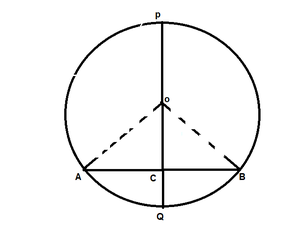According to the question,

Prove: PQ bisects ∠AOB

Proof:

Form the given figure, we get

PQ is a diameter of circle which bisects the chord AB at C.

In ΔBOC and ΔAOC

OC = OC [Common side]

AC = BC [Given]

By SSS condition

ΔAOC ≅ ΔBOC

Hence, BY C.P.C.T

∠AOC = ∠BOC

So, PQ bisects ∠AOB

Hence proved.

### Question 8. Prove that two different circles cannot intersect each other at more than two points.

Solution:

Prove: two different circles cannot intersect each other at more than two points.

Proof:

Let us considered the two circles intersect in three points, i.e., A, B and C.

Now as we know that, points A, B, and C are non-collinear.

So, a unique circle passes through these three points(i.e., A, B, C).

Now, this is a contradiction to the fact that the two given circles are passing through A, B, C.

Hence, two circles cannot intersect each other at more than two points.

Hence, proved.

### Question 9. A line segment AB is of length 5cm. Draw a circle of radius 4 cm passing through A and B. Can you draw a circle of radius 2 cm passing through A and B? Give reason in support of your answer.

Solution:

According to the question, given that

A line segment AB = 5 cm,

One circle having radius r1 = 4 cm which is passing through point A and B

and other circle having radius r2 = 2 cm.

As we know that the largest chord of any circle is equal to the diameter of that circle.

So, 2 × r2 < AB

Hence, there is no possibility to draw a circle whose diameter is smaller than the length of the chord.

### Question 10. An equilateral triangle of side 9 cm is inscribed in a circle. Find the radius of the circle.

Solution: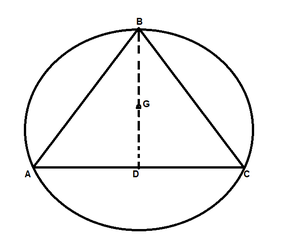According to the question,

Let us considered ABD is the equilateral triangle and the side of the triangle is 9cm

and AD is one of its median.

Find: the radius of the circle

So,

Let us assume G be centroid of ΔABC

Then AG:GD = 2:1

As we know that in an equilateral triangle, the centroid coincides with circumcentre,

So, G is centre of circumference with circumradius GA.

Also, G is the centre and GD ⊥ BC,

Now we find the radius(AG) of the circle

= 3√3 cm

Hence, the radius of the circle is 3√3 cm

### Question 11. Given an arc of circle, complete the circle.

Solution: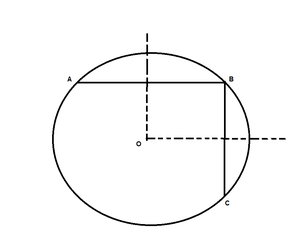Steps of Construction:

Step 1: Let us considered three points i.e., A, B and C on the given arc

Step 2: Join AB and BC

Step 3: Now draw the perpendicular bisectors of chords AB and BC which intersect each other at point O.

So, the centre of the circle is O.

Step 4: Now join OA

Step 5: Hence, the centre of the circle is O, so the radius of the circle is OA.

Hence, the complete the circle.

### Question 12. Draw different pairs of circles. How many points does each pair have in common? What is the maximum number of common points?

Solution: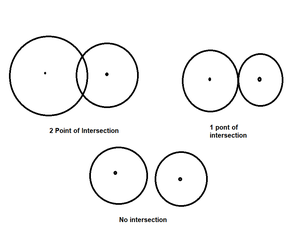Here, the first set of circles contains 2 common points, Second set of circles contains 1 common points, and

third set of circles contains 0 common points.

Hence, the maximum number of common points are 2.

### Question 13. Suppose You are given a circle. Give a construction to find its centre.

Solution:Steps Of Construction:

Step 1: Let us considered three points i.e., A, B and C on the given circle.

Step 2: Join AB And BC.

Step 3: Now draw the perpendicular bisectors of chord AB and BC which intersect each other at point O.

Step 4: As we know that the perpendicular bisectors of chord always pass through the centre, so the centre of the circle is point O.

### Question 14. Two chords AB and CD of lengths 5cm and 11cm respectively of a circle are parallel to each other and are the opposite side of its centre. If the distance between AB and CD is 6cm, find the radius of the circle.

Solution: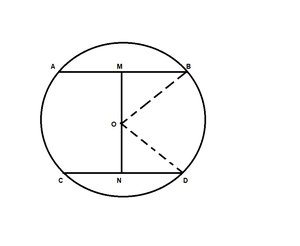According to the question, given that

The length of chod AB = 5cm

The length of chod CD = 11cm

The distance between AB and CD(i.e., MN) = 6cm

Find: the radius of the circle

So, draw OM ⊥ AB and ON ⊥ CD

Now, join OB and OD

As we know that, perpendicular from centre to chord bisects the chord

So, BM = AB/2 = 5/2

ND = CD/2 = 11/2

Let us assume ON be x, so OM will be 6 – x.

In ΔMOB,

OM2 + MB2 = OB2

(6 – x)2 + (5/2)2 = OB2

36 + x2 – 12x + 25/4 = OB2  ………(i)

In ΔNOD,

ON2 + ND2 = OD2

x2 + (11/2)2 = OD2

x2 + 121/4 = OD2  ………(ii)

We have OB = OD (radii of same circle)

So from eq(i) and (ii), we get

36 + x2 – 12x +25/4 = x2 +121/4

12x = 36 + 25/4 – 121/4

12x = 48/4

x = 1

Now put the value of x in eq(i), we get

OD2 = 1 + 121/4

OD = 5√5/2

Hence, the radius of circle is 5√5/2

### Question 15. The lengths of two parallel chords of a circle are 6cm and 8cm. If the smaller chord is at a distance of 4cm from the centre, what is the distance of the other chord from the centre?

Solution: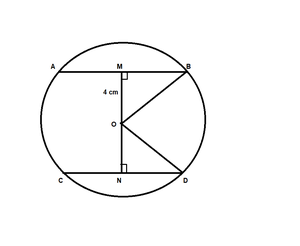According to the question, given that

The length of chod AB = 6cm

The length of chod CD = 8cm

The distance between the chord AB and the centre(OM) = 4 cm

Find: The distance between the chord CD and the centre(ON)

So, MB = AB/2 = 6/2 = 3cm

In ΔOMB,

OM2 + MB2 = OB2

42 + 92 = OB2

OB = 5cm

In ΔOND,

OD = OB = 5cm [radii of same circle]

ND = CD/2 = 8/2 = 4cm

ON2 + ND2 = OD2

ON2 + 42 = 52

ON2 = 25 – 16

ON = 3cm

Hence, The distance between the chord CD and the centre(ON) is 3cm.

Attention reader! Don’t stop learning now. Join the First-Step-to-DSA Course for Class 9 to 12 students , specifically designed to introduce data structures and algorithms to the class 9 to 12 students

My Personal Notes arrow_drop_up